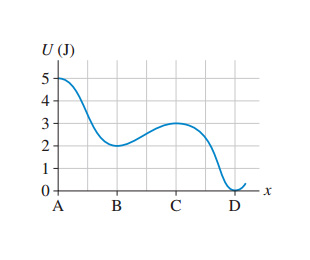# Problem: The following image is the potential-energy diagram for a 500 g particle that is released from rest at A.(a) What is the particles speed at B?(b) What is the particles speed at C?(c) What is the particles speed at D?

###### FREE Expert Solution
79% (421 ratings)
###### Problem Details

The following image is the potential-energy diagram for a 500 g particle that is released from rest at A.
(a) What is the particles speed at B?
(b) What is the particles speed at C?
(c) What is the particles speed at D?Frequently Asked Questions

What scientific concept do you need to know in order to solve this problem?

Our tutors have indicated that to solve this problem you will need to apply the Force & Potential Energy concept. You can view video lessons to learn Force & Potential Energy. Or if you need more Force & Potential Energy practice, you can also practice Force & Potential Energy practice problems.

How long does this problem take to solve?

Our expert Physics tutor, Julia took 3 minutes and 17 seconds to solve this problem. You can follow their steps in the video explanation above.

What professor is this problem relevant for?

Based on our data, we think this problem is relevant for Professor Calvin's class at LEHMAN-CUNY.